Risk

# How to Use Excel to Sell Gelato

A simple sales scenario provides an example of how Excel can be used to run regression analyses — and boost rainy-day revenue.

A reader asks a question that calls for using Excel and a multiple regression. She writes, “I run a gelato stand.  After 10 days of sales, I discovered that each day, I would either make a lot of money or nearly go broke.  As I analyzed sales, I began to feel that temperature and rain might be two important determining factors in how much money I make. On rainy or cool days, fewer people buy gelato.

I set up a table (see Figure 1), to show each day’s sales, temperature, and whether it rained. Based on the data I’ve collected, how can I determine the relationship between sales, temperature, and rainfall?”

Fig. 1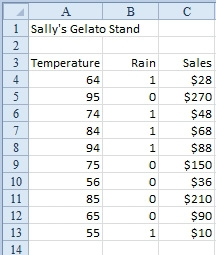Solution: You need to do a multiple regression, after which you will have a formula that predicts sales like this:
Y=m1x1 + m2x2 + b
Sales = TemperaturexM1 + Rain x M2 + b

Excel’s LINEST function can return the values M1, M2, and b that best describe your sales model. Here’s how to run through the steps:

1. LINEST is going to return three values, so select a range of three cells that are side by side. The first argument is the range of known sales figures. The second argument is the range of temperatures and rainfall.
2. Press Ctrl+Shift+Enter to calculate the array formula (Figure 2).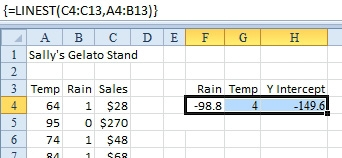Fig. 2

3. Enter a prediction formula in column D to see how well the regression calculation describes sales. The results seem so-so. The prediction in D6 is right on the mark. The predictions in D11 and D12 are off by \$20 each — an error of 10% (Figure 3).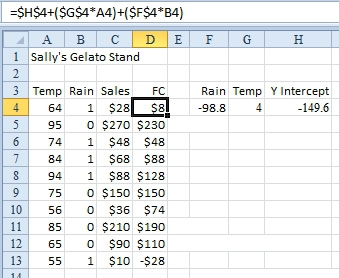Fig. 3

4. To get the additional statistics that LINEST can return to show how well the results match reality, add a fourth argument: TRUE. Be sure to enter the function in a five-row range (Figure 4).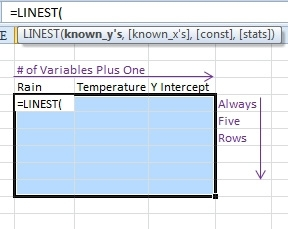Fig. 4

5. Press Ctrl+Shift+Enter. You will get the results shown in Figure 5.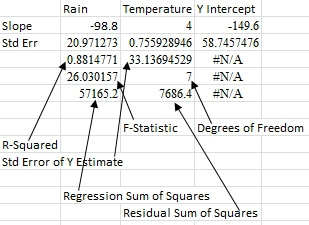Fig. 5

I only somewhat paid attention in statistics class, but I know a key statistical indicator is the R-Squared value. It ranges from 0 to 1, where 1 is a perfect match, and 0 is a horrible match. The 0.88 value here confirms that the prediction model is pretty good but not perfect.

Additional Details: Regression models try to force actual results into a straight-line formula. The fact is that life may not fit in a straight-line formula. Because I created the spreadsheet used here, I know the actual data in the gelato model uses the formula (Temperature – 50) x \$2 if raining and (Temperature – 50) x \$6 if not raining. In this example, the gelato-stand owner was correct that ice cream sales are dependent on rain and temperature, but even a powerful regression engine could not predict the absolutely correct formula.

CFO contributor Bill Jelen is an Excel MVP and the author of 35 books about Microsoft Excel.